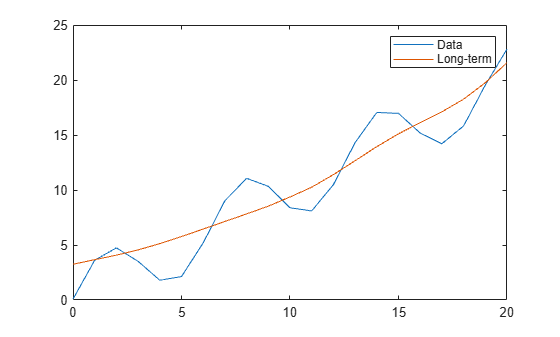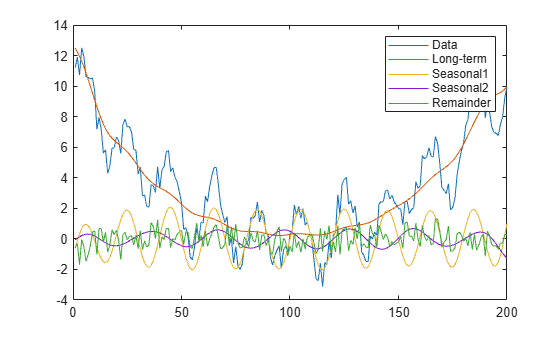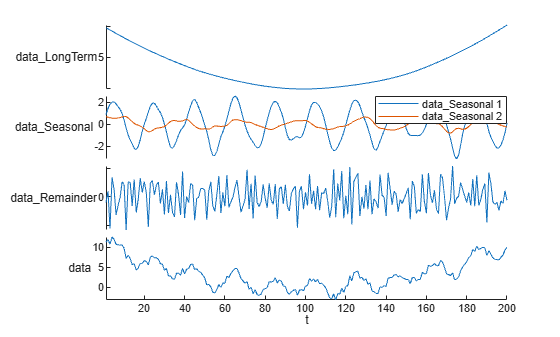# trenddecomp

Find trends in data

## Syntax

``LT = trenddecomp(A)``
``LT = trenddecomp(A,"ssa",lag)``
``LT = trenddecomp(___,NumSeasonal=n)``
``LT = trenddecomp(A,"stl",period)``
``[LT,ST,R] = trenddecomp(___)``
``D = trenddecomp(T)``
``D = trenddecomp(T,"ssa",lag)``
``D = trenddecomp(T,___,NumSeasonal=n)``
``D = trenddecomp(T,"stl",period)``

## Description

example

````LT = trenddecomp(A)` finds trends in a vector of data using singular spectrum analysis (SSA), which assumes an additive decomposition of the data such that `A = LT+ST+R`. In this decomposition, `LT` is the long-term trend in the data, `ST` is the seasonal, or oscillatory, trend (or trends), and `R` is the remainder. `LT` is a vector with the same length as `A`.SSA is a useful algorithm when the periods of the seasonal trends are unknown. The SSA algorithm assumes that the input data is uniformly spaced.```
````LT = trenddecomp(A,"ssa",lag)` also uses the SSA algorithm to find trends in `A` and additionally specifies a lag value, which determines the size of the matrix on which the singular value decomposition is computed, as described in . Larger values of `lag` typically result in more separation of the trends.The value of `lag` must be a scalar in the interval [3,N/2] where N is the length of `A`. If the period of the seasonal trend is known, then specify `lag` as a multiple of the period.```
````LT = trenddecomp(___,NumSeasonal=n)` specifies the number of seasonal trends to return, using any of the input argument combinations in the previous syntaxes.```
````LT = trenddecomp(A,"stl",period)` finds the trends in `A` using the STL algorithm, which is an additive decomposition based on a locally weighted regression, as described in . STL requires a period for the seasonal trend. When the data has only one seasonal trend, specify `period` as a scalar value. For multiple seasonal trends, specify `period` as a vector whose elements are the periods for each seasonal trend.The STL algorithm assumes that the input data is uniformly spaced.```

example

````[LT,ST,R] = trenddecomp(___)` also returns the seasonal trends and the remainder from the decomposition. The lengths of `ST` and `R` match the length of the input vector `A`. When `A` contains multiple seasonal trends, `ST` is a matrix whose columns contain each seasonal trend.```
````D = trenddecomp(T)` finds trends in a table or timetable of data using SSA. `trenddecomp` operates on each table variable separately. `D` is a table or timetable whose variables contain the long-term trend, seasonal trends, and remainder for each variable. `trenddecomp` returns multiple seasonal trends as one variable in `D`, whose columns contain each seasonal trend.```
````D = trenddecomp(T,"ssa",lag)` specifies an SSA lag value for table or timetable input and applies the lag to all table variables.```
````D = trenddecomp(T,___,NumSeasonal=n)` specifies the number of seasonal trends when you use SSA on table or timetable data, and applies the number to each table variable.```

example

````D = trenddecomp(T,"stl",period)` finds trends using STL with the specified seasonal trend periods. `trenddecomp` applies the periods to each table variable.```

## Examples

collapse all

Compute the long-term trend in a vector of data using singular spectrum analysis.

Create a vector of data `A` that contains a long-term trend, seasonal trend, and noise component.

```t = (0:20)'; A = 3*sin(t) + t + 0.1*rand(21,1);```

Compute the long-term trend. Plot the data and the long-term trend.

```LT = trenddecomp(A); plot(t,A,t,LT) legend("Data","Long-term")```Decompose a vector of data into its long-term trend, two seasonal trends, and remainder using singular spectrum analysis.

Create a vector of data `A` that contains a long-term trend, two seasonal trends with different periods, and a noise component.

```t = (1:200)'; trend = 0.001*(t-100).^2; period1 = 20; period2 = 30; seasonal1 = 2*sin(2*pi*t/period1); seasonal2 = 0.75*sin(2*pi*t/period2); noise = 2*(rand(200,1) - 0.5); A = trend + seasonal1 + seasonal2 + noise;```

Decompose the data using the SSA algorithm. Plot the data, the trends, and the remainder.

```[LT,ST,R] = trenddecomp(A); plot([A LT ST R]); legend("Data","Long-term","Seasonal1","Seasonal2","Remainder")```Decompose tabular data into its long-term trend, two seasonal trends, and remainder using the STL algorithm.

Create a table `T` whose variable `data` contains a long-term trend, two seasonal trends with different periods, and a noise component.

```t = (1:200)'; trend = 0.001*(t-100).^2; period1 = 20; period2 = 30; seasonal1 = 2*sin(2*pi*t/period1); seasonal2 = 0.75*sin(2*pi*t/period2); noise = 2*(rand(200,1) - 0.5); data = trend + seasonal1 + seasonal2 + noise; T = table(data)```
```T=200×1 table data ______ 11.204 11.896 10.722 12.502 11.939 10.646 10.57 10.479 10.527 9.6793 7.1756 7.9505 7.1704 5.6206 5.8256 4.2817 ⋮ ```

Decompose the data using the STL algorithm. Plot the data, trends, and remainder.

```D = trenddecomp(T,"stl",[20 30]); D = addvars(D,data); stackedplot(D) xlabel("t")```## Input Arguments

collapse all

Input array, specified as a numeric vector.

Data Types: `single` | `double`

Lag value for SSA, specified as a numeric scalar in the interval [3,N/2] where N is the length of the input data. Larger values of `lag` typically provide more separation of the trends. If the period is known, then specify `lag` as a multiple of the period.

Number of seasonal trends for SSA, specified as an integer scalar larger than 0.

Period for STL, specified as a numeric scalar, numeric vector, `duration` scalar, or `duration` vector. When the data has one seasonal trend, specify `period` as a scalar. When the data has multiple seasonal trends, specify `period` as a vector whose elements are the periods for each seasonal trend. `period` can have type `duration` for timetable input data only.

Tabular input data, specified as a table or timetable. `trenddecomp` operates on each table variable separately. Timetables must be uniformly spaced.

## Output Arguments

collapse all

Long-term trend, returned as a vector with the same length as the input vector.

Seasonal trend, returned as a vector or matrix. `ST` is a vector when the data has one seasonal trend and a matrix when the data has multiple seasonal trends, with each column corresponding to one seasonal trend. The number of rows in `ST` matches the length of the input vector.

Remainder, returned as a vector with the same length as the input vector.

Trends, returned as a table or timetable when the input data is a table or timetable. `D` contains the long-term trend, seasonal trends, and remainder of the decomposition as separate table variables. For multiple seasonal trends, the number of columns in the resulting variable of `D` matches the number of seasonal trends.

 Golyandina, Nina, and Anatoly Zhigljavsky. Singular Spectrum Analysis for Time Series. SpringerBriefs in Statistics. Berlin, Heidelberg: Springer Berlin Heidelberg, 2013. https://doi.org/10.1007/978-3-642-34913-3.

 Cleveland, R.B., W.S. Cleveland, J.E. McRae, and I. Terpenning. “STL: A Seasonal-Trend Decomposition Procedure Based on Loess.” Journal of Official Statistics 6 (1990): 3–73.